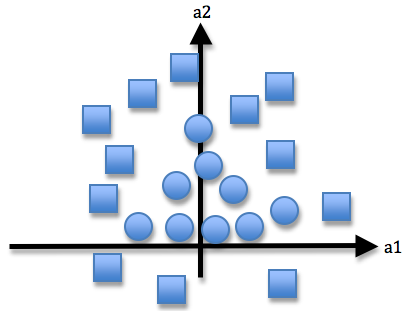2 views

I have a supervised learning problem where my algorithm will be given a set of training examples for learning whether a shape is a circle of a square. I was wondering which type of ANN would be the best. I know that you can choose a perceptron if the data is linearly separable... Surely I can easily have a hyperplane that divides my squares and circles up? So isn't a perceptron a good enough choice? However, aren't multilayer feedforward networks more commonly used? What is the natural choice and why?

The following image shows the training data given to the system. The NN needs to classify two-dimensional data A=[a1,a2] into squares and circles.Thank you.

by (108k points)

The answer to your question depends upon the data representation and your data is not linearly separable. Thus, a perceptron is not appropriate for this data. You can use a multi-layer perceptron which can be used more often because they can do everything a single-layer perceptron can do (look up universal approximation theorem). A radial-basis function is also applicable to this solution.

You can also refer the following link for a better understanding of the criteria for choosing the best neural network: https://pdfs.semanticscholar.org/5d6e/f0074069de335742cabdc01c350715e9888e.pdf